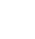# Split text by hard returns in a cell

If you have a dataset in Excel or has perhaps been copied over from Word where multiple lines appear in a single Excel cell, it can be difficult to split the text out into a useable format. The example below was used in a recent project for a client who needed not only to split out the data, but sort it according to data type (Vehicle Registration / Telephone etc) and present it in a table format on another worksheet.

The example code below shows how to run through all the data in Sheet1 and output the splits into a table on a worksheet called ‘Output’. The text to split is in column D and the original data is also cleaned up at the end as well as scanning the output for cells where the original data has placed the Vehicle reg and telephone number in the incorrect tcells.

This was possible to run on over 27,500 rows of data and gave an instant output for the client to manage with their team of agents. We’d be happy to help sort your data or advise on the best way to achieve your data objective. Feel free to contact us using the form below or leave a comment and we’ll get back to you.

``` Private Sub Split_Cell_by_Hard_returns() Sub Button2_Click() With ActiveSheet lastrow = .Cells(.Rows.Count, "A").End(xlUp).Row End With For n = 5 To lastrow Dim str() As String If Len(ActiveSheet.Range("D" & n).Value) Then ' CHECK IF THE ACTIVE CELL IS NOT EMPTY. ' SPLIT THE ACTIVE CELL'S VALUE WITH LINE FEED (vbLf). str = VBA.Split(ActiveSheet.Range("D" & n).Value, vbLf) ' REARRANGE TEXT TO MULTIPLE COLUMNS. ActiveSheet.Range("D" & n).Resize(1, UBound(str) + 1).Offset(0, 1) = str End If Next n For r = 5 To lastrow For c = 5 To 15 Worksheets("Output").Cells(r - 3, c - 4) = Worksheets("Sheet1").Cells(r, c) Next c Next r With Worksheets("Output") lastrow = .Cells(.Rows.Count, "A").End(xlUp).Row End With For x = 2 To lastrow If IsNumeric(Left(Worksheets("Output").Range("B" & x), 1)) Then b = Worksheets("Output").Range("B" & x).Value c = Worksheets("Output").Range("C" & x).Value Worksheets("Output").Range("B" & x) = c Worksheets("Output").Range("C" & x) = b b = "" c = "" End If Next x ```

```Worksheets("Sheet1").Range("E:L").Clear End Sub ```